# Trigonometry : Sec, Csc, Ctan

## Example Questions

### Example Question #1 : Sec, Csc, Ctan

Find the value of the trigonometric function in fraction form for triangle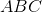.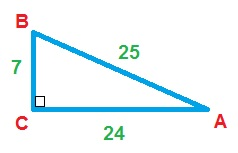What is the secant of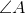?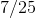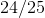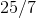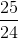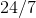Explanation:

The value of the secant of an angle is the value of the hypotenuse over the adjacent.

Therefore: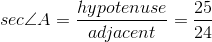### Example Question #11 : Trigonometric Operations

Which of the following is the equivalent to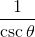?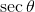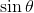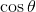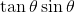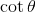Explanation:

Since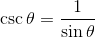: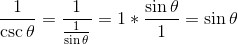### Example Question #641 : Sat Subject Test In Math I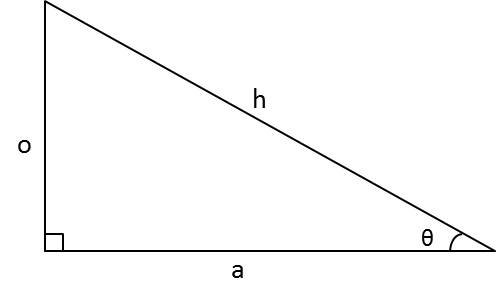For the above triangle, what is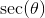if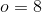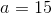and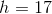?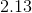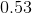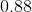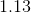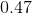Explanation:

Secant is the reciprocal of cosine.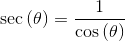It's formula is: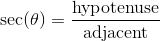Substituting the values from the problem we get,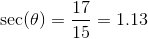### Example Question #1 : Sec, Csc, CtanFor the above triangle, what is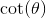if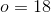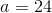and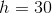?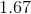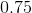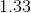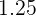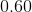Explanation:

Cotangent is the reciprocal of tangent.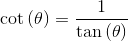It's formula is: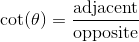Substituting the values from the problem we get,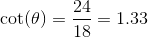### Example Question #2 : Sec, Csc, Ctan

Determine the value of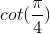.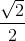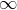Explanation:

Rewritein terms of sine and cosine.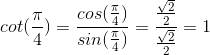### Example Question #3 : Sec, Csc, Ctan

Evaluate: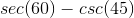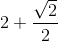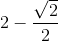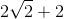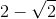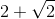Explanation:

Evaluate each term separately.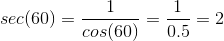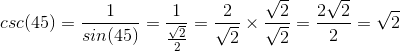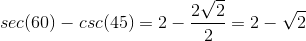### Example Question #21 : Trigonometric Operations

Pick the ratio of side lengths that would give sec C.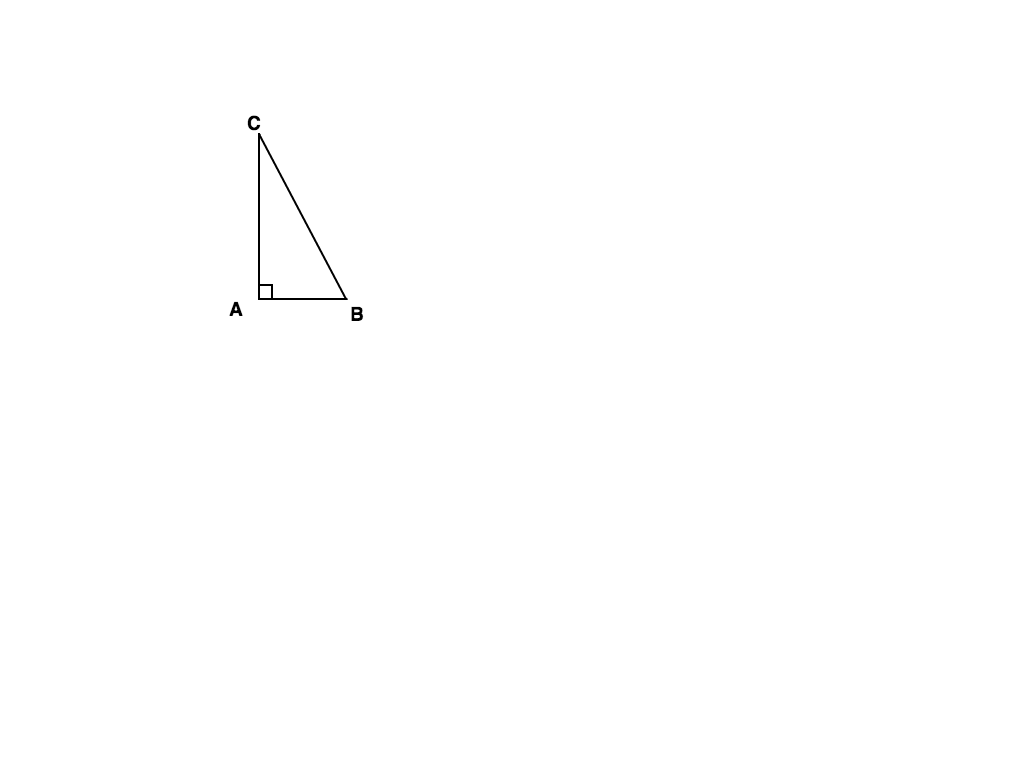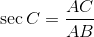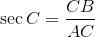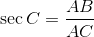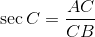Explanation: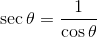Find the ratio of Cosine and take the reciprocal.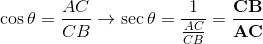### All Trigonometry Resources